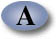UNIFIED ENGINEERING Fall 2004

Ian A. Waitz

Problem T4 (Unified Thermodynamics)

Consider a thermodynamic system containing air at v1=1 m3/kg, p1=100 kPa. The system is compressed to 0.5 m3/kg via anyone of three quasi-static processes: isobaric, isothermal, or adiabatic. Assume that cp = 1.0035 kJ/kg-K, cv = 0.7165 kJ/kg-K, and R = 0.287 kJ/kg-K. (LO#4, LO#5)

a) Sketch all three processes on the same p-v diagram.

b) For each process determine the pressure and temperature at the final state.

c) For each process determine the work done by the system and the heat transferred to the system.

d) For each process calculate the change in enthalpy of the air.